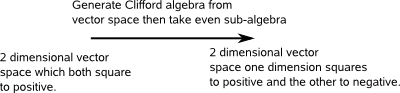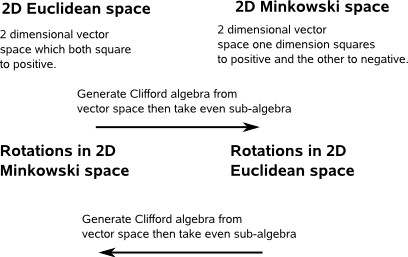# Maths - Using 2D Multivectors for Rotation

In order to work with rotations using the multiplication operator, in particular to combine two individual rotations to give an equivalent single rotation, then we need to use the even sub-algebra.## Complex Numbers

So if we start with 2D euclidean space, that is two dimensions that both square to positive, then we get the Clifford algebra G 2,0,0:

e = scalar (squares to positive)
e1 = vector (squares to positive)
e2 = vector (squares to positive)
e12 = bivector (squares to negative)

Taking the even sub-algebra we take just the scalar and bivector dimensions:

e = scalar (squares to positive)
e12 = bivector (squares to negative)

This is equivalent (isomorphic to) complex number algebra.

## Double Numbers

This time we start with a vector space where one dimension squares to positive and the other squares to negative, then we get the Clifford algebra G 1,1,0:

e = scalar (squares to positive)
e1 = vector (squares to positive)
e2 = vector (squares to negative)
e12 = bivector (squares to positive)

Taking the even sub-algebra we take just the scalar and bivector dimensions:

e = scalar (squares to positive)
e12 = bivector (squares to positive)

This is equivalent (isomorphic to) double number algebra.

## Duality

So, from the above, there appears to be a duality between Euclidean and Minkowski space, where rotations in Euclidean space are calculated using Minkowski space and visa versa.Where I can, I have put links to Amazon for books that are relevant to the subject, click on the appropriate country flag to get more details of the book or to buy it from them.Clifford Algebra to Geometric Calculus: A Unified Language for Mathematics and Physics (Fundamental Theories of Physics). This book is intended for mathematicians and physicists rather than programmers, it is very theoretical. It covers the algebra and calculus of multivectors of any dimension and is not specific to 3D modelling.